Plotting Spatial Derivatives of the Magnetic Field

March 5, 2014

Being able to compute the spatial gradients of the magnetic field or magnetic flux density is needed in areas such as radiology, magnetophoresis, and geophysics. One of the most important applications is in the design of magnetic resonance imaging machines, where it’s important to analyze not only the field strength, but also the spatial variation of the field. Today’s blog post demonstrates how to compute and plot the gradients of the magnetic field in 3D electromagnetic simulations in COMSOL Multiphysics.

Background and Objective

Whenever you are computing the magnetic fields induced by currents or currents induced by time-varying magnetic fields, as well as 3D electromagnetic wave problems, COMSOL Multiphysics uses so-called vector (or curl, or Nedelec) elements. The vector element is also used in 2D and 2D axisymmetric magnetic field simulations that involve in-plane current distribution. In these cases, the vector element computes the magnetic vector potential, \mathbf{A}. However, the vector element can only compute the first derivative of the field.

The magnetic flux density, \mathbf{B}, magnetic field, \mathbf{H}, and the magnetic vector potential can be related via the magnetic permeability, \mathbf{\mu}, using the following equations:

\mathbf{B=\nabla \times A}
\mathbf{B=\mu H}

The equations show that the magnetic flux density and the magnetic field are functions of the first derivative of the magnetic vector potential. Since the second derivative is not defined on vector (curl) elements, the spatial gradients of \mathbf{B} and \mathbf{H} cannot be computed.

The technique demonstrated here shows how each component of the magnetic field \mathbf{H}=[Hx,Hy,Hz] can be mapped to a separate variable by adding an extra equation that has three unknown variables. These new variables use the Lagrange element. Since both first and second order spatial derivatives are defined on Lagrange elements, it now becomes possible to obtain the spatial gradients of the \mathbf{B} and \mathbf{H} fields.

Note that, when solving for 2D or 2D-axisymmetric magnetic field problems involving out of plane currents, or static magnetic field problems without any current flow within the model, the Lagrange elements are used to solve the governing equations, and these do make the second spatial derivative available. The method shown in this post applies only to cases where the vector elements are used to compute the fields.

Step-by-Step Tutorial Using a Helmholtz coil

Next, let’s look at an example where we can demonstrate the technique introduced above. We will see how you can compute and plot the spatial derivatives of the magnetic field produced by a Helmholtz coil. A detailed description of this model along with step-by-step instructions to simulate the coil can be found in the Model Gallery. A Helmholtz coil is a pair of parallel circular coils separated by a distance of one radius where the current flows through both the coils in the same direction. Some known applications of this kind of coil are used for canceling the earth’s magnetic field and generating controlled magnetic fields for experiments.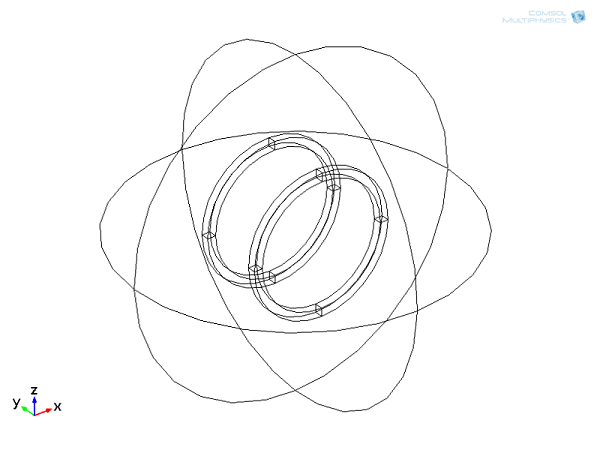Geometry of the coils together with the air domain.

This model uses the Magnetic fields physics interface and the Multiturn coil domain feature to model the two coils. Each coil has 10 turns and 0.25 mA current circulating through it.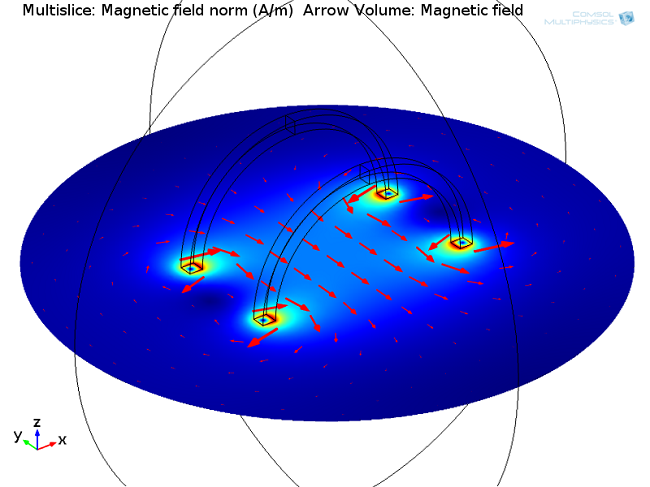Plot of the magnitude of the magnetic field (A/m) and an arrow plot showing the direction of the magnetic field vector.

Plotting the Gradients of the Magnetic Field

We will start with the solved example from the Model Gallery and see how we can compute the derivatives of the magnetic field.

The first step is to map the results of the magnetic field on Lagrange elements. For that, we add the Coefficient Form, PDE interface with three dependent variables (one per component), which uses the Lagrange element by default. We also set the right units for the dependent variables.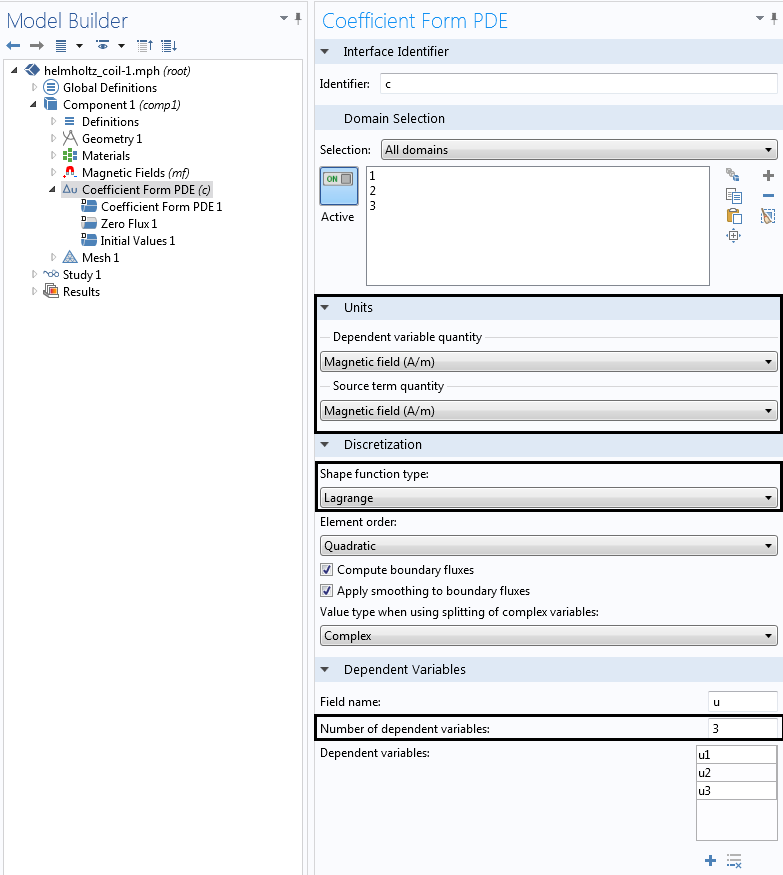Definition of the units and number of variables.

The second step is to correctly set up the coefficients of the PDE, in order to get the right expression for mapping the results.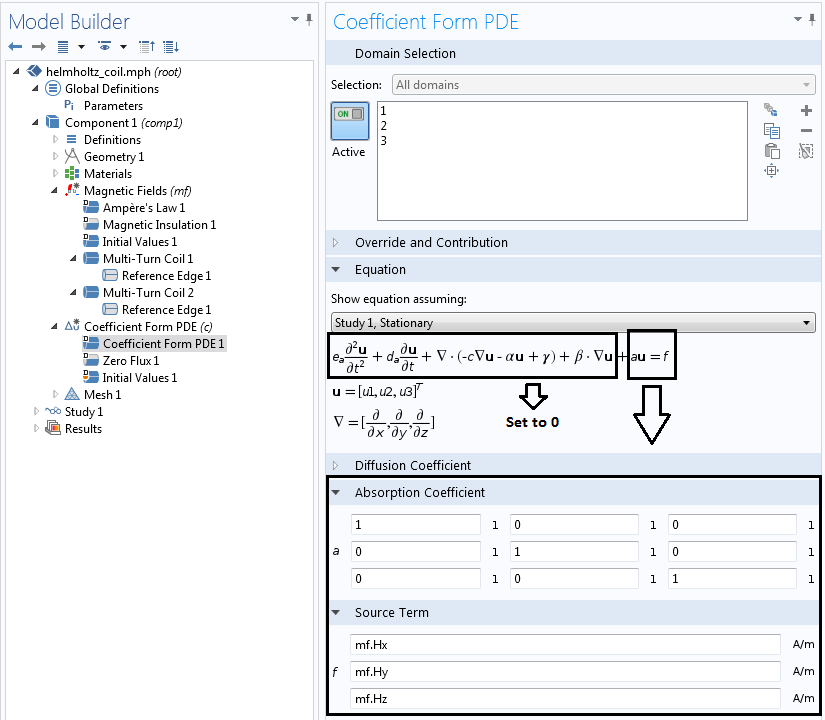Definition of the PDE settings.

Here we set all coefficients other than \text{a} and \mathbf{f} to 0, thereby creating an equation with the following form:

\text{a}\mathbf{u} = \mathbf{f}

Where \text{a} = 1, \mathbf{u} is the vector field, and \mathbf{f} represents the components of the magnetic field. Hence we can directly relate these new variables to the components of the magnetic field vector, that is:

u1 = mf.Hx
u2 = mf.Hy
u3 = mf.Hz

Concerning the study setup, we first compute only the Magnetic fields problem in Study 1, and in the second study we compute the variables u1, u2, and u3. In order to take the values from the electromagnetic solution, we activate the feature “Values of variables not solved for” using the study settings shown below.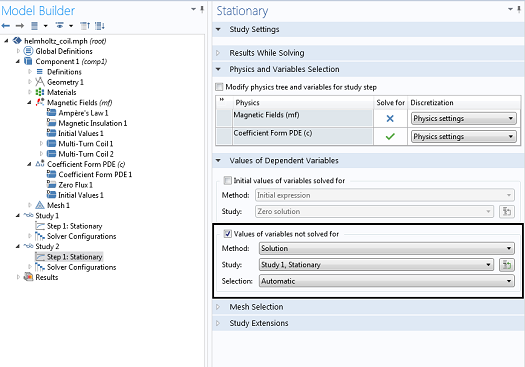Definition of the study steps.

In order to compute the gradients of the magnetic field, we append to the variable name the spatial direction in which we want to to compute the partial derivative. For example, u1x would compute the gradient of the x-component of the magnetic field with respect to the x-direction.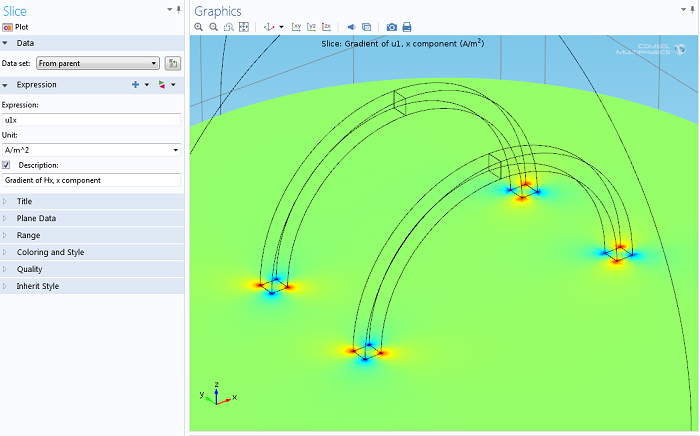Plot of the gradient (with respect to the x-direction) of the x-component of the magnetic field.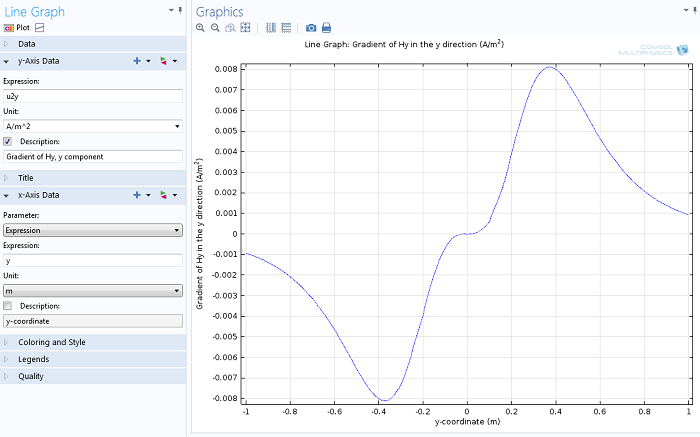Line plot on the centerline along y-coordinate of the gradient (with respect to the y-direction) of the y-component of the magnetic field

CategoriesTuan-Anh Le
July 2, 2016

But when I checked it, I see u1 and mf.x is different. Plz expain it. Thank youTuan-Anh Le
July 2, 2016

But when I checked it, I see u1 and mf.Hx is different. Plz expain it. Thank youRahmetullah Cagil
October 2, 2017

I applied a similar procedure for magnetic flux density and just like Tuan-Anh, I see that mf.Bx and u1 are different, they are almost identical in shape but u1 is scaled down by three orders of magnitude. What could be the cause for this error?Tijani Aziz Feki
October 10, 2017

Hi all
I did all steps about mf.Bx but the results from second study is different from what we can obtain from first study, what is the reason and how should I fix this problem?
thanks.Caty Fairclough
October 13, 2017

Hi Tuan-Anh, Rahmetullah, and Tijani

Online Support Center: https://www.comsol.com/support
Email: support@comsol.comkai zhang
November 9, 2017

We alse can select the “dode” interface and set “f=u1-mf.Hx” and so on. It seemed this way will be more accurate for “u1” in the result for the transient study, such as “ecore transformer.mph” in the library.Simon Houis
August 7, 2018

Hello,

Thank you very much for this interesting post. I managed to compute the gradient of a stationary study (1 single current applied). Now I would like to do the same but for a frequency sweep. I computed a model over 10 frequencies and I ‘d like now to compute spatial gradients for each frequency. The idea is vizualize on a single plot the magn gradient over x for all frequencies. Nonetheless when I applied the method you described, I am only able to vizualise results for the last frequency and not all of them.

Could you tell me how to proceed to compute gradient for all frequencies?

Regards.Caty Fairclough
August 7, 2018

Hi Simon,

Thanks for your interest in this blog post!

For your questions, I would suggest contacting our Support team.

Online Support Center: https://www.comsol.com/support
Email: support@comsol.com

EXPLORE COMSOL BLOG